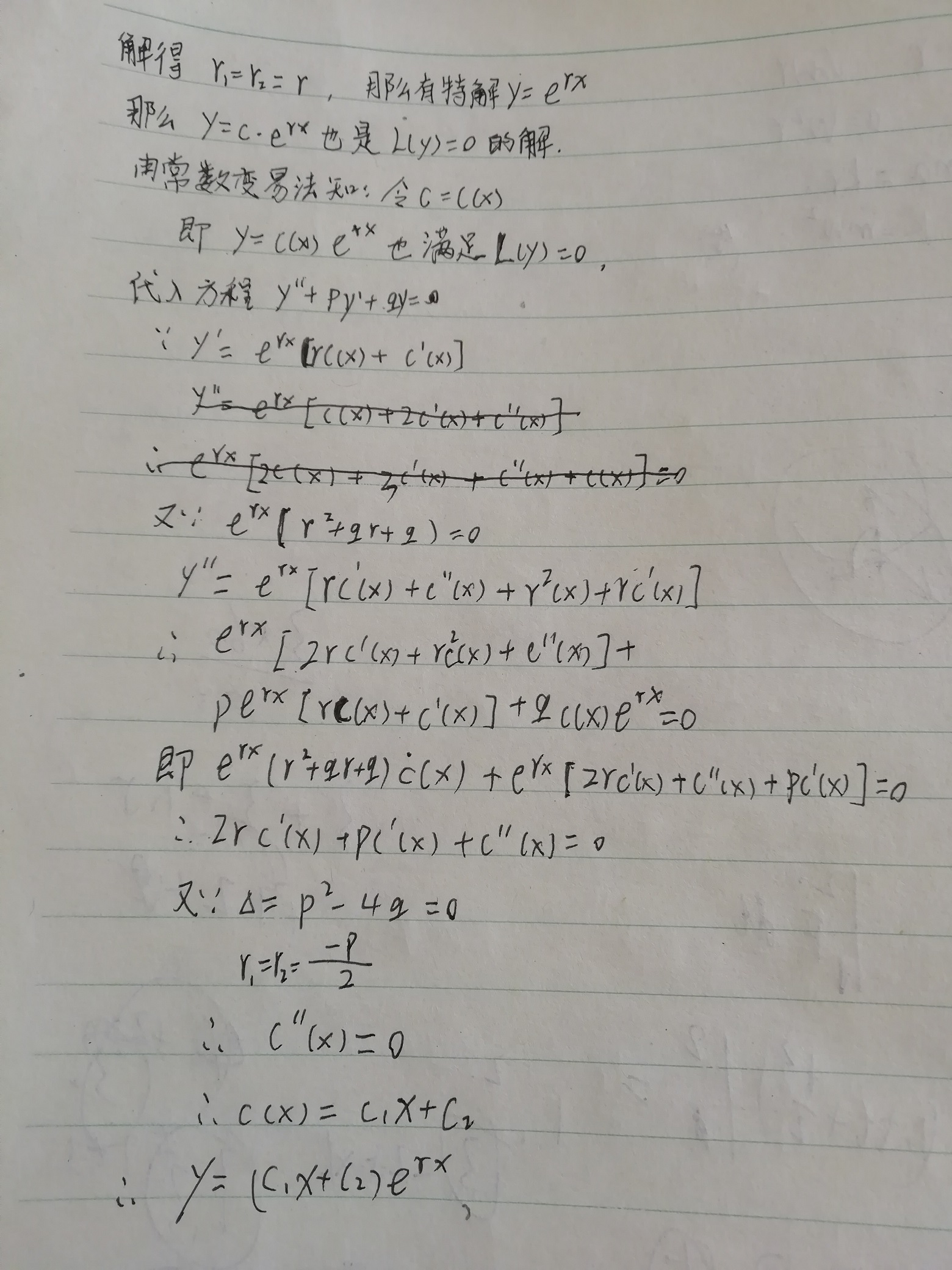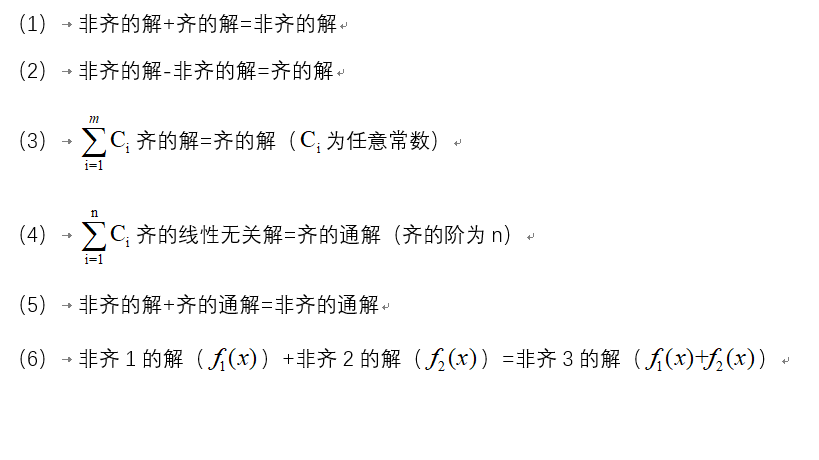• ## 二阶线性微分方程

千次阅读 2019-02-27 23:02:30
二阶线性微分方程定义：二阶线性微分方程定义：二阶线性微分方程定义： 形如： y′′+P(x)y′+Q(x)y=f(x)y&amp;#x27;&amp;#x27; + P(x)y&amp;#x27; + Q(x)y = f(x)y′′+P(x)y′+Q(x)y=f(x)的方程称为...
二阶线性微分方程
一. 认识二阶线性微分方程

$二阶线性微分方程定义：$
形如： $y'' + P(x)y' + Q(x)y = f(x)$的方程称为二阶线性微分方程
当 $f(x)=0$恒成立时，称该方程为二阶线性齐次方程；
当 $f(x)\neq0$时，称该方程为二阶线性非齐次方程。

$定理：$
$若y_1，y_2是二阶线性齐次方程y'' + P(x)y' + Q(x)y = 0 的解，则y=c_1y_1+c_2y_2（c_1,c_2 \in R)仍是它的解。$

二.  二阶线性微分方程求解思路
$【观察发现】y=c_1y_1+c_2y_2（c_1,c_2 \in R)$ $在形式上存在两个常数，符合高阶微分方程通解的规律。$ $（即n阶微分方程的通解中存在n个常数）$
$【提出问题(一）】$$是否能够认为对任意y_1,y_2，存在y=c_1y_1+c_2y_2（c_1,c_2 \in R)为$y’’ + P(x)y’ + Q(x)y = 0$的通解?$
$【找到反例】 当y_1=x, y_2=kx 满足y'' + P(x)y' + Q(x)y = 0 时，$
$发现y=c_1y_1+c_2y_2=c(x+kx) = cx 中，只存在一个常数，$
$不再符合高阶微分方程通解的规律。$
$\Longrightarrow$ $对任意y_1,y_2，存在y=c_1y_1+c_2y_2（c_1,c_2 \in R)为y'' + P(x)y' + Q(x)y = 0的通解假设错误$
$【提出问题(二）】$ $当y_1,y_2满足什么关系是，能够使y=c_1y_1+c_2y_2（c_1,c_2 \in R)为y'' + P(x)y' + Q(x)y = 0的通解成立？$

$函数组的线性相关性:$
$设有函数组y_1,y_2....y_n,（其中y是关于x的函数） \forall x\in I,$$如果存在一组不全为零的常数k_1,k_2...k_n,$$使得k_1y_1+k_2y_2+...+k_ny_n=0 ,$ $则称函数组y_1,y_2....y_n在区间I上时线性相关的;$
$如果只有当k_1=k_2=...k_n=0时成立，$$则称函数组y_1,y_2....y_n在区间I上时线性无关的。$

$【观察发现】当y_1,y_2线性无关时y=c_1y_1+c_2y_2始终存在两个常数，$$满足高阶微分方程通解的规律。$
$【提出问题(三）】如何找到二阶线性齐次方程y'' + P(x)y' + Q(x)y = 0 的两个线性无关的解？$

$线性无关的判定方法：$
$(1) y_1,y_2是一个函数组，若存在k \in R，满足y_1=ky_2,$
$则y_1,y_2线性相关，否则线性无关。$
$(2) y_1,y_2....y_n是一个函数组，若存在I=1，2...n,y_i=0,$ $则函数组y_1,y_2....y_n线性相关。$
$(3)函数组：部分相关\rightarrow 整体相关$  ;  $整体不相关\rightarrow 部分不相关$.

$【提出问题(四）】若已知二阶线性齐次方程y'' + P(x)y' + Q(x)y = 0的一个解，能否找到与其线性无关的另一个解？$
（使用与推导一阶线性非齐次方程相似的常数变易法进行求解）
$假设 :y_1 是y'' + P(x)y' + Q(x)y = 0的一个解，$
$y_2=C_{(x)}y_1为y'' + P(x)y' + Q(x)y = 0的另一个解$(在求证过程中，始终认为C是关于x的函数
$\Rightarrow$       $y_2'=C'y_1+Cy_1'$
$y_2''=C''y_1+2C'y'+Cy''$       $代入y'' + P(x)y' + Q(x)y = 0$
$\Rightarrow$       $C''y_1+[ 2y' + P(x)y ]C'=0$
$令u= C' ,$         $u'=C''$
$\Rightarrow$       $u'y_1+[ 2y' + P(x)y ]u=0$               $(分离变量)$
$\Rightarrow$       $\int \frac{1}{u}du = -\int \frac{2y_1+P(x)y_1}{y_1}dx$
$lnu=ln\frac{1}{y_1^2}-\int P(x)dx +lnC_1$
$又\because 只需求得y'' + P(x)y' + Q(x)y = 0的一个与y_1线性无关的特解，$                  $可以令C_1=1$
$\Rightarrow$       $u=\frac{1}{y_1^2}e^{-\int P(x)dx }$
$\Rightarrow$       $C=\int \frac{1}{y_1^2}e^{-\int P(x)dx} dx+C2$
$令C_2=0$
$\Rightarrow$      $\frac{y_1}{y_2}=\int \frac{1}{y_1^2}e^{-\int P(x)dx} dx$
$\therefore$      $y_2=y_1\int \frac{1}{y_1^2}e^{-\int P(x)dx} dx$

【刘维尔公式】
$若y_1 是y'' + P(x)y' + Q(x)y = 0的一个解，$ $则方程另一个与y_1线性无关的特解:$
$y_2=y_1\int \frac{1}{y_1^2}e^{-\int P(x)dx} dx$


展开全文• 二阶常系数线性微分方程通解： 首先来观察方程的形式：y"+py’+qy=0，由前面的第二条定理可知，方程的通解的形式一定是C1y1+C2y2，将这个通解代入原方程的话，我们会发现它和它的一阶导、它的二阶导都相差常数倍，...
先占个坑，明天来填。。。。明天能有时间吗？？？？但是我把写博客这个事情必须坚持下去，等闲下来的时候一定要留几篇存稿。
+++++++++++++++++++2020年3月20日08点27分+++++++++++++++++++
一.定理：
首先要认识到二阶线性齐次微分方程的解满足的性质，值得注意的是这不仅仅是待会儿要提到的二阶线性常系数齐次微分方程满足，对于一般的二阶线性齐次微分方程也是满足的。
1.叠加原理：有限个方程的解的线性组合仍然是方程的解。
这个原理是由于方程的线性的性质所以决定的，如果有线性代数的基础，那么对于这个性质就很好理解了，就算没有，也应该很好理解。L(y1)=0,L(y2)=0,（至于L是什么意思的话，那么请参见（9/300）对于线性微分算子的描述），由于线性(也就是说y是一次的)，L(y1)+L(y2)=L(D)y1+L(D)y2=L(D)(y1+y2)=L(y1+y2)=0,所以y1+y2也是方程的解，在推广到有限个解就ok了。
2.方程的通解:如果y1，y2是方程线性无关的两个特解的话，y1和y2的线性组合的全体就是方程的通解。
对于这句话的理解，首先要明白什么是通解，通解，首先它是方程的解，但是这个解它拥有和方程阶数相等的不定的常数，比如C1y1+C2y2，如果y1和y2线性无关的话，那么这样的一个组合构成的一个空间就是方程的通解了。其实这个也很好理解，如果y1和y2线性相关的话，那么他们的线性组合一定能够用Cy1来表示，这就和方程的阶数二不相等了，既然不相等，那就不能算是通解。
3.复值根：当P（x）和Q（x）都是实值函数，对于复值根而言，他的实部、虚部、共轭都是方程的根，（一带三，斗地主的充值玩家都不敢这么玩）。对于这个定理的话，emmmm，作为一个工科学生，，，，，emmm，不会。（希望能在评论区看到通俗易懂的理解，/龇牙）
二.二阶常系数线性微分方程的通解：
首先来观察方程的形式：y"+py’+qy=0，由前面的第二条定理可知，方程的通解的形式一定是C1y1+C2y2，将这个通解代入原方程的话，我们会发现它和它的一阶导、它的二阶导都相差常数倍，这不🈲让人联想到erx,它正好满足这个条件，那么我们就只需要就求得y1=er1x和y2=er2x即可，然后我们发现代入原方程的形式是(r2+pr+q)erx=0,这正好可以消去erx，得到特征方程r2+pr+q=0,而对于这个方程的解的话，就会有三种情况两个异值实根、两个同值实根、一对共轭复值根，分别对应于一元二次方程的根的判别式＞、=、＜0的情况。
下面来看看实根的两种情况，复值根的情况会在后面的欧拉方程那一节再提到的。
r1≠r2:C1er1x+C2er2x
这个其实就很好理解了，er1x、er2x分别是两个特解，根据解的性质写出来就好了。
r1=r2:(C1+C2x)er1x
至于这个我就把推导过程写一下。有两行求导求错了，请忽视。这里其实就是用了一个常数变易法，对于一个线性方程，右端为0，在等式的左右边同时乘以一个因式，等式仍然成立。


展开全文• 一、线性微分方程的结构 1.1 二阶齐次线性方程 y′′+P(x)y′+Q(x)y=0(1) y''+P(x)y'+Q(x)y=0 \tag{1} y′′+P(x)y′+Q(x)y=0(1) 定理1：如果函数y1(x)y_1(x)y1​(x)与y2(x)y_2(x)y2​(x)是方程（1）的两个，...
一、线性微分方程的解的结构
1.1 二阶齐次线性方程
$y''+P(x)y'+Q(x)y=0 \tag{1}$
定理1：如果函数$y_1(x)$与$y_2(x)$是方程（1）的两个解，那么
$y=C_1y_1(x)+C_2y_2(x) \tag{2}$
也是方程（1）的解，其中$C_1,C_2$是任意常数。
解（2）从形式上看含有$C_1$和$C_2$两个任意常数，但它不一定是方程（1）的通解。那么在什么情况下（2）式才是方程（1）的通解呢？要解决这个问题，还得引入新概念，即函数组的线性相关与线性无关。
设$y_1(x),y_2(x),···,y_n(x)$为定义在区间$I$上的n个函数，如果存在n个不全为零的常数$k_1,k_2,···,k_n$，使得当$x\in I$时有恒等式
$k_1y_1+k_2y_2+···+k_ny_n=0$
成立，那么称这n个函数在区间I上线性相关；否则线性无关。
应用上述概念可知，对于两个函数的情形，它们线性相关与否，只要看它们的比是否为常数；如果比为常数，那么它们就线性相关；否则就线性无关。
定理2：如果$y_1(x)$与$y_2(x)$是方程（1）的两个线性无关的特解，那么
$y=C_1y_1(x)+C_2y_2(x)$
就是方程（1）的通解，$C_1,C_2$是任意常数。
推论：如果$y_1(x),y_2(x),···，y_n(x)$是n阶齐次线性方程
$y^{(n)}+a_1(x)y^{(n-1)}+···+a_{n-1}(x)y'+a_n(x)y=0$
的n个线性无关的解，那么，此方程的通解为
$y=C_1y_1(x)+C_2y_2(x)+···+C_ny_n(x)$
其中$C_1,C_2,···,C_n$为任意常数。
1.2 二阶非齐次线性方程
一阶非齐次线性微分方程 的通解由两部分构成：一部分是对应的齐次方程的通解，另一部分是非齐次方程本身的一个特解。实际上，不仅一阶非齐次线性方程的通解具有这样的结构，而且二阶及更高阶的非齐次线性微分方程的通解也具有同样的结构。
定理3：设$y^*(x)$是二阶非齐次线性方程
$y''+P(x)y'+Q(x)y=f(x) \tag{3}$
的一个特解。$Y(x)$是与（3）对应的齐次方程（1）的通解，则
$y=Y(x)+y^*(x)$
是二阶非齐次线性方程（3）的通解。
由于对应的齐次方程（1）的通解$Y=C_1y_1+C_2y_2$中含有两个任意常数，所以$y=Y+y^*$中也含有两个任意常数，从而它就是二阶非齐次线性方程（3）的通解。
定理4：设非齐次线性方程（3）的右端$f(x)$是两个函数之和，即
$y''+P(x)y'+Q(x)y=f_1(x)+f_2(x)$
而$y_1^*(x)$与$y_2^*(x)$分别是方程
$y''+P(x)y'+Q(x)y=f_1(x)$
与
$y''+P(x)y'+Q(x)y=f_2(x)$
的特解，则$y_1^*(x)+y_2^*(x)$就是原方程的特解。
这已订立通常称为线性微分方程的解的叠加原理。
定理3和定理4也可推广到n阶非齐次线性方程。
二、 常数变易法
为解一阶非齐次线性方程，我们用了常数变易法。这方法的特点是：如果$Cy_1(x)$是齐次线性方程的通解，那么，可以利用变换$y=uy_1(x)$（这变换是把齐次方程的通解中的任意常数C换成未知函数$u(x)$而得到的）去解非齐次线性方程。这一方法也适用于解高阶线性方程。下面就二阶线性方程来作讨论。
2.1 已知齐次方程的两个解$y_1(x)$和$y_2(x)$
如果已知齐次方程（1）的通解为
$Y(x)=C_1y_1(x)+C_2y_2(x)$
那么，可以用如下的常数变易法去求非齐次方程（3）的通解。令
$y=y_1(x)v_1+y_2(x)v_2 \tag{4}$
要确定未知函数$v_1(x)$及$v_2(x)$使（4）式所表示的函数满足非齐次方程（3）。为此对（4）式求导，得
$y'=y_1v_1'+y_2v_2'+y_1'v_1+y_2'v_2$
由于两个未知函数$v_1,v_2$只需使（4）式所表示的函数满足一个关系式（3），所以可规定它们再满足一个关系式。从$y'$的上述表示可看出，为了使$y''$的表示式中不含$v_1''$和$v_2''$，可设
$y_1v_1'+y_2v_2'=0 \tag{5}$
从而
$y'=y_1'v_1+y_2'v_2$
再求导，得
$y''=y_1'v_1'+y_2'v_2'+y_1''v_1+y_2''v_2$
把$y,y',y''$代入方程（3），化简得
$y'_1v'_1+y_2'v'_2+(y_1''+Py_1'+Qy_1)v_1+(y_2''+Py_2'+Qy_2)v_2=f$
注意到$y_1$及$y_2$是齐次方程（1）的解，故上式即为
$y_1'v_1'+y_2'v_2'=f \tag{6}$
联立方程（5）与（6），在系数行列式
$W =\begin{vmatrix} y_1 & y_2 \\ y_1'& y_2' \end{vmatrix} =y_1y_2'-y_1'y_2 \neq 0$
时，可解得
$v_1'=-\frac{y_2 f}{W},\, v_2'=\frac{y_1f}{W}$
对上两式积分（假定$f(x)$连续），得
$v_1=C_1+\int(-\frac{y_2f}{W})dx,\, v_2=C_2+\int\frac{y_1f}{W}dx$
于是得非齐次方程（3）的通解为
$y=C_1y_1+C_2y_2-y_1\int\frac{y_2f}{W}dx+y_2\int\frac{y_1f}{W}dx$
2.2 已知齐次方程的一个解$y_1(x)$
如果只知齐次方程（1）的一个不恒为零的解$y_1(x)$，那么，利用变换$y=uy_1(x)$，可把非齐次方程（3）化为一阶线性方程。
事实上，把
$y=y_1u,\,y'=y_1u'+u_1'u,\, y''=y_1u''+2y_1'u'+y_1''u$
代入方程（3），化简得
$y_1u''+(2y_1'+Py_1)u'+(y_1''+Py_1'+Qy_1)u=f$
由于$y_1''+Py_1'+Qy_1=0$，故上式为
$y_1u''+(2y_1''+Py_1)u'=f$
令$u'=z$，上式即化为一阶线性方程
$y_1z'+(2y_1'+Py_1)z=f \tag{7}$
把方程（3）化为方程（7）以后，按一阶线性方程的解法，设求得方程（7）的通解为
$z=C_2Z(x)+z^*(x)$
积分得$u=C_1+C_2U(x)+u^*(x)$（其中$U'(x)=Z(x),u^{*}{’}(x)=z^*(x)$），
上式两端乘$y_1(x)$，便得方程（3）的通解
$y=C_1y_1(x)+C_2U(x)y_1(x)+u^*(x)y_1(x)$
上式方法显然也适用于求齐次方程（1）的通解。


展开全文• 二阶线性齐次微分方程 为 齐 二阶线性非齐次微分方程 为 非齐 重要的性质、定理（共6条）： 证明1：若y1、y2、y3是非齐的，a、b、c为常数且a+b+c=0，y=a y1+by2+cy3则y是齐的 由(7) ，则需要证明a y1+by...
以下简称

二阶线性齐次微分方程 为 齐
二阶线性非齐次微分方程 为 非齐

重要的性质、定理（共6条）：证明1：若y1、y2、y3是非齐的解，a、b、c为常数且a+b+c=0，y=a y1+by2+cy3则y是齐的解

由(7)  ，则需要证明a y1+by2+cy3是 齐的解。

因为y1、y2、y3是非齐的解，故y1－y2、y2-y3是齐的解，则
C1（y1－y2）+C2（y2-y3）也是齐的解
故C1（y1－y2）+C2（y2-y3）就是齐的解
显然y1的系数=C1=a
y2的系数=-C1+C2=b
y3的系数=-C2=c
a+b+c=0

证明2： 若y1、y2、y3是非齐的解，a、b、c为常数且a+b+c=１，y=a y1+by2+cy3 则y是非齐的解

由（1），则需要证明a y1+by2+cy3是非齐的解＋齐的解

因为y1、y2、y3是非齐的解，故y1－y2、y2-y3是齐的解，则
C1（y1－y2）+C2（y2-y3）也是齐的解，而y3是非齐的解，
故C1（y1－y2）+C2（y2-y3）+y3就是非齐的解
显然y1的系数=C1=a
y2的系数=-C1+C2=b
y3的系数=-C2+1=c
a+b+c=1

证明3：若y1、y2、y3是非齐的线性无关解，a、b、c为任意常数且a+b+c=0，

y=a y1+by2+cy3，则y是齐的通解
由（7）
y1、y2、y3是非齐的线性无关解，现假设y1-y2、y2-y3也是线性无关的，
则齐的通解y=C1(y1-y2)+C2(y2-y3)
显然y1的系数=C1=a
y2的系数=-C1+C2=b
y3的系数=-C2=c
a+b+c=0

补充证明：现证明y1-y2、y2-y3是线性无关的。

假设线性有关，则存在不全为0的k1和k2，
使得k1（y1-y2）+k2（y2-y3）恒等于0
即：
(k1)y1+(k2-k1)y2-(k2)y3恒等于0
由于y1、y2、y3是线性无关的，故k1=0、k2=0，与假设相违背。因此，y1-y2、y2-y3是线性无关的。

证明4：若y1、y2、y3是非齐的线性无关解，a、b、c为任意常数且a+b+c=1，y=a y1+by2+cy3，则y是非齐的通解

由（5）
y1、y2、y3是非齐的线性无关解，现假设y1-y2、y2-y3也是线性无关的，
则齐的通解y=C1(y1-y2)+C2(y2-y3) ，
非齐的解为y3（你换成y1或者y2都是一样的，因为最后大家系数都是要加在一起的）
显然y1的系数=C1=a
y2的系数=-C1+C2=b
y3的系数=-C2+1=c
a+b+c=1
展开全文• *本文略去了很多证明，只记录结论 *文中的微分方程均指代二阶常...由于是二阶线性微分方程，所以它有两个，记为y1、y2y1、y2y_1、y_2，若y1y2≠Cy1y2≠C\frac{y_1}{y_2} \neq C(即两个之比不为常数)，则y1、...高等数学
• 微分方程的通解 = 对应的二阶常系数齐次线性微分方程通解 + 自身的一个特解 简单记为：通解 = 齐次通解 + 特解。 二阶常系数齐次线性微分方程通解的解法：二阶常系数齐次线性微分方程的通解 下面只需要解出微分方程...
• 介绍了求解二阶常系数非齐次线性微分方程的2种简易方法———降阶法和积分法,扩大了可求解二阶常系数非齐次线性微分方程的范围,并举例说明了它们的应用.
• §2.1 二阶微分方程2.1 Second Order Equations微分方程 MIT公开课《微分方程线性代数》 2.1 二阶微分方程​v.youku.com二阶微分方程的应用特别广泛，因为它包含了加速度项，即速度的导数——二阶导数 。...
• 二阶线性微分方程就是指形如y′′+py′+q=0y&#x27;&#x27;+py&#x27;+q=0y′′+py′+q=0的微分方程，一种解法就是使用二阶微分方程的公式，这里介绍另一种方法。 对于二阶微分方程，如果找到两个线性...微分方程
• (光看一遍书很快就又忘了,在此记录一下) y′′+py′+qy=0y''+py'+qy=0y′′+py′+qy=0 ...第三步:根据特征方程的两个根的不同情形,按照下列表格写出微分方程通解: 特征方程r2+pr+q=0r^2+pr+q=...
• 这三类方程的形状很特殊，它们是二阶线性微分方程的三个典型代表。 一般形式的二阶线性微分方程之间的共性和差异，往往可以从对这三类方程的研究中得到。 一维弦振动方程是双曲型的，一维热传导方程是抛物...
• 利用两类Riccati方程z′=z2 - a(x)z+ b( x)的求解公式,给出了两类二阶线性微分方程通解,应用这些只与方程系数a(x)与b( x)相关的求解公式,求通解过程十分简捷.
• 在假设二阶变系数非齐次线性微分方程两个变系数关系已知的前提下，利用降阶法推出几类二阶变系数齐次线性微分方程通解表达式.
• 人生有无数的可能性，考研的结果一定不是终点!但做的每一个选择都要坚持到最后!这是对自己、对梦想最大的尊重!用探索方法代替消极迷茫，用寻求技巧抵消杂乱慌张!争分夺秒，竭尽所能!悉心浇灌，静候花开!...数学
• 一般二阶线性非齐次微分方程与对应齐次方程的的关系@(微积分)设p(x),q(x),f(x),f(x)≠0p(x),q(x),f(x),f(x)\neq 0为连续函数，对于下面的二阶线性非齐次方程：y″+p(x)y′+q(x)y=f(x)y''+p(x)y'+q(x)y = f(x) ...
• 主要介绍了二阶常系数非齐次线性微分方程通解与特解
• 二阶常系数微分方程通解 (一.) 二阶常系数微分方程通解的组成： 其对应二阶常系数微分方程通解 + 二阶常系数微分方程的特解 (二.) 构造二阶常系数微分方程的特解 形如：y′′+py′+qy=Pm(x)eαxy&amp;...
• 一、二阶线性微分方程的引入 【例1】设有一弹簧，它的上端固定，下端挂一个质量为的物体。当物体处于静止状态时，作用在物体上的重力与弹性力大小相等，方向相反。这个位置就是物体的平衡位置。如图，取轴铅直向下...高等数学 微分方程
• 这里以二阶线性微分方程为例。 形如方程5的称为二阶线性微分方程。   线性的概念定义为：   下面讨论 二阶线性微分方程，这些性质也可以推广到n阶线性方程：   1. 线性微分方程的的结构  目前，式（7...
• 一般地，n个自变量的二阶线性微分方程可表示为 ∑i,j=1naij(x1,⋅⋅⋅,xn)∂2u∂xi∂xj+∑j=1nbj(x1,⋅⋅⋅,xn)∂u∂xj+c(x1,⋅⋅⋅,xn)u=f(x1,⋅⋅⋅,xn)(1) \sum_{i,j=1}^na_{ij}(x_1,···,x_n)\frac{\partial...
• :证明二阶齐次线性方程通解可以表示成任意两个线性无 86 关解的线性组合}} \setlength\epigraphwidth{ 0.7 \linewidth} 87 \epigraph{汝当更求古之哲王以为师,如吾,不足法也.夫取法于上,仅得其中;取法 88...
• 好难啊，第十期还没有更，十一期的也没时间了，明天满课，还有个视频要做，再占个坑，如果计划顺利，11期是二阶线性非齐次的微分方程。嗯，加油。 ...
• 对于任意的二元二阶齐次线性微分方程， \(a_{11}\dfrac{\partial^2u}{\partial x^2}+2a_{12}\dfrac{\partial^2 u}{\partial x\partial y}+a_{22}\dfrac{\partial^2 u}{\partial y^2}+b_1\dfrac{\...
• 形如下面形式的微分方程叫做二阶线性微分方程： 若f(x)=0,称其为齐次的，否则是非齐次的。 先来看二阶微分方程的一些性质，我们可以把这些性质推广到n阶（至少先人已经做到了）。 对于此方程： 方程1 1.1如果y1...
• 二阶常系数线性方程通解反推方程@(微积分)引例是这样的： 设cosxcosx与xexxe^x为某n阶常系数线性齐次方程的两个解，则最小的n = ？，相应的首项系数为1的方程是？ 分析：由cosx是一个解，则必有另一解sinx,±i\......